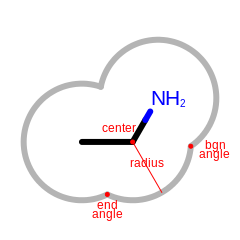# OESurfaceArc¶

class OESurfaceArc


This class represents OESurfaceArc that stores data for drawing the arcs of molecule surface. See Figure: Example of the data stored in the OESurfaceArc class.Example of the data stored in the OESurfaceArc class

Note

Angles are interpreted such that $$0.0^{\circ}$$ and $$360.0^{\circ}$$ degrees are at the 12 o’clock position, $$90.0^{\circ}$$ degree corresponds to 3 o’clock, etc.

## Constructors¶

OESurfaceArc()


Default constructor.

OESurfaceArc(const OEDepict::OE2DAtomDisplay *adisp,
const OEDepict::OE2DPoint &center, double bgnAngle, double endAngle,


Creates an OESurfaceArc object with the given parameters.

OESurfaceArc(const OESurfaceArc &rhs)


Copy constructor.

## operator=¶

OESurfaceArc &operator=(const OESurfaceArc &rhs)


Assignment operator.

## GetAtomDisplay¶

const OEDepict::OE2DAtomDisplay *GetAtomDisplay() const


Returns the pointer of the OE2DAtomDisplay object the given arc belongs to.

## GetBgnAngle¶

double GetBgnAngle() const


Returns the angle (in degrees) where the arc of the surface starts.

## GetCenter¶

const OEDepict::OE2DPoint &GetCenter() const


Returns the (x,y) coordinates of the center of the surface arc.

## GetEndAngle¶

double GetEndAngle() const


Returns the angle (in degrees) where the arc of the surface ends.

double GetRadius() const


Returns the radius of the arc.

## SetAtomDisplay¶

bool SetAtomDisplay(const OEDepict::OE2DAtomDisplay *adisp)


Sets the atom display to which the arc belongs.

## SetBgnAngle¶

bool SetBgnAngle(double angle)


Sets the angle where the arc of the surface begins.

angle

The angle has to in the range of $$[0.0^{\circ}, 360.0^{\circ}]$$

## SetCenter¶

bool SetCenter(const OEDepict::OE2DPoint &center)


Sets the (x,y) coordinates of the center of the surface arc.

## SetEndAngle¶

bool SetEndAngle(double angle)


Sets the angle where the arc of the surface ends.

angle

The angle has to in the range of $$[0.0^{\circ}, 360.0^{\circ}]$$

bool SetRadius(double radius)